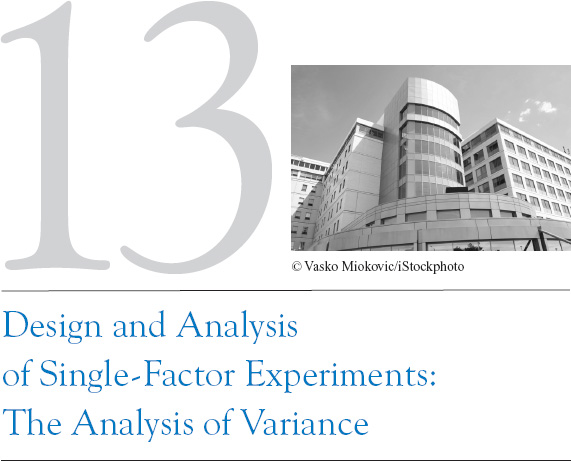Experiments are a natural part of the engineering and scientific decision-making process. Suppose, for example, that a civil engineer is investigating the effects of different curing methods on the mean compressive strength of concrete. The experiment would consist of making up several test specimens of concrete using each of the proposed curing methods and then testing the compressive strength of each specimen. The data from this experiment could be used to determine which curing method should be used to provide maximum mean compressive strength.

If there are only two curing methods of interest, this experiment could be designed and analyzed using the statistical hypothesis methods for two samples introduced in Chapter 10. That is, the experimenter has a single factor of interest—curing methods—and there are only two levels of the factor. If the experimenter is interested in determining which curing method produces the maximum compressive strength, the number of specimens to test can be determined from the operating characteristic curves in Appendix Chart VII, and the t-test can be used to decide if the two means differ.

Many single-factor experiments require that more than two levels of the factor be considered. For example, the civil engineer may want to investigate five different curing methods. In this chapter we show how the analysis of variance (frequently abbreviated ANOVA) ...

Get Applied Statistics and Probability for Engineers, 5th Edition now with the O’Reilly learning platform.

O’Reilly members experience books, live events, courses curated by job role, and more from O’Reilly and nearly 200 top publishers.